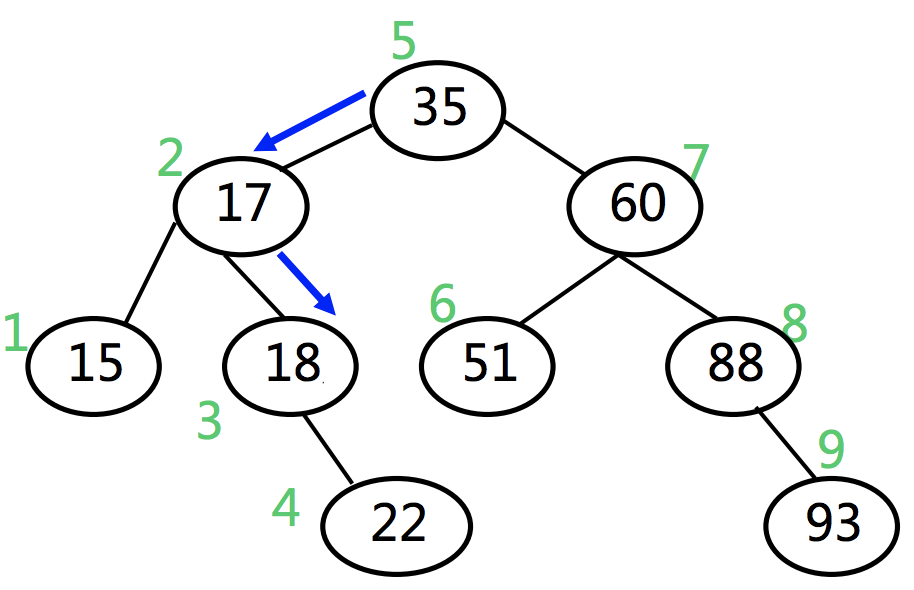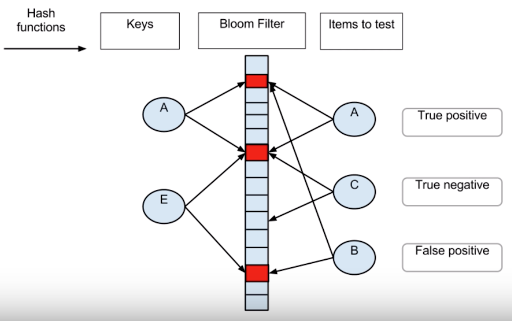# 哈希表

## 搜索概述

1. 预排序

排序算法本身比较耗时，无法实时来进行，因此排序只能作为预处理手段，在检索之前完成

2. 建索引

如果对某种数据需要反复查找，则需要建立比较高效的索引，检索时充分利用辅助索引信息。代价是牺牲一定的空间，以空间换时间。同时维护成本也有所提高，当数据变化时，索引也要改变

3. 散列

所谓散列就是把数据组织到一个表中，将Query通过某种算法(hash)得出它的value在表中的位置，从而完成检索。这种方式的优点是查询时间为O(1)，缺点是不适合进行范围查询，一般也不允许出现重复的关键码。另外，散列也不适合对磁盘文件进行检索（可以选择 B 树方法）。

### 顺序检索

1. 检索成功/失败

假设每个关键码等概率出现: $P_i=1/n$，从后向前遍历，如果最后一个数字恰好为关键码，则只需比较一次，如果关键码在第一个数字的位置，则需要比较$n-1$次，因此检索成功的概率为$p$，检索失败的概率为${1-p}$：

2. 平均检索长度 将$p$,$q$带入公式可得到 $(n+1)/2 < ASL < (n+1)$## 散列搜索

1. 根据关键码值，直接找到记录的存储地址
2. 不需要把待查关键码与候选记录集合的某些记录进行逐个比较

1. 有一个散列函数h,以结点的关键码K为自变量,函数值h(K)作为结点的存储地址，Address = Hash(key)
2. 需要一个存储空间，通常是一个一维数组，散列地址是数组的下标

key:        1       32      43      24      95      86      77      108     249     10
hashCode:   1       2       3       4       5       6       7       8       9       0
hashMap:    <1,1>   <2,32>  <3,43>  <4,24>  <5,95>  <6,86>  <7,77>  <8,108> <9,249> <0,10>


1. hashCode冲突如何处理(例如32,42)
2. 数据多了，散列表大小怎么调整
3. key的分布情况
4. value的检索频率，例如对检索频率较高的key放到基地址，等等

### 散列函数的选取

1. 确定性：同一关键码总是被映射到同一地址
2. 快速：O(1)
3. 满射：尽可能充分的覆盖整个散列空间
4. 均匀：关键码映射到散列表中各个位置的概率尽量接近，也就是要将所有可能的key尽量均匀的压缩到散列空间，避免局部汇聚的情况

• 除余法

1. 不动点: 无论$M$如何选取，总有hash(0)= 0,这和所有key等概率映射的条件相矛盾
2. 零阶均匀: 设key的取值范围从[0，R)，平均分配到M个桶，则相邻的key的散列地址也必然相邻。我们希望能找到更高阶的均匀，即相邻的key在散列后的位置不再相邻

Knuth认为：A 可以取任何值，与待排序的数据特征有关。一般情况下取黄金分割最理想

• 平方取中法

hash( 123 ) = 512 // 123^2 = 19 ，保留中间三位做散列地址
hash( 1234567 ) = 556 //1234567^2 = 1524177489，保留中间三位做散列地址

• 折叠法

1. 移位叠加 — 把各部分的最后一位对齐相加
2. 分界叠加 — 各部分不折断，沿各部分的分界来回折叠（zigzag order），然后对齐相加，将相加的结果当做散列地址
//移位叠加                          //分界叠加
key = 123456789                    key = 12345678

123                            123
456                            654
+   789                        +   789
-----------                    -------------
hash(123456789) = 1368          hash(123456789) = 1566

• 多项式法

static size_t hashCode(char s[]){ //近似多项式计算，用位运算代替乘法运算
int h=0;
for(size_t n = strlen(s), i=0; i<n; i++){
h = (h<<5) | (h>>27); //交换h的前5个bit和后27个bit
h += (int)s[i];
}
return (size_t)h;
}


### 冲突解决

• Chaining

//使用数组
k1 : [v1,v1'v1'',...]
k2 : [v2,v2'v2'',...]
...
kn : [vn,vn',vn'',...]


1. 如果使用数组的方式，该预留多少个槽位给冲突的元素呢？
2. 无论预留多少，极端情况下仍然可能不够用1. 线性试探

所谓线性试探是指，当插入或者查找某个value时，一旦hashCode发生冲突，则试探其（基地址）后面的单元（探查序列），其试探的方式为key生成一个散列地址序列$d_1,d_2,…,d_{m-1}$(所有$d_i (0<i<m$ 是后继散列地址)

 //基地址：
hash(key) % M

//试探地址：
d1 = [hash(key) + 1] % M
d2 = [hash(key) + 2] % M
d3 = [hash(key) + 3] % M
...
dn = [hash(key) + n] % M


具体来说，以存储为例（查找的步骤类似）：

1. 首先根据其散列函数计算hashcode(基地址)
2. 根据基地址先找到对应的存储位置（内存位置），如果是空的，则放入
3. 如果发该位置已被占用（冲突），则重新计算hashCode，向后不断试探，直到找到一个空桶放入。

无论是插入还是查找，都假定每个关键码的探查序列中至少有一个存储位置是空的作为结束标记，否则可能会进入死循环。接下来看一个具体的查找的例子：

 M = 15, hash(key) = key % 13

key  : | 26 | 25 | 41| 15| 68| 44| 6 |   |   |   | 36 |    | 38 | 12 | 51 |
index: | 0  |  1 | 2 | 3 |  4| 5 | 6 | 7 | 8 | 9 | 10 | 11 | 12 | 13 | 14 |
-------|----|----|---|---|---|---|---|---|---|---|----|----|----|----|----|
value: | x  |  y | z | a | b | c | d |   |   |   |  e |    |  f |  g | h  |


上述例子中

2. 平方试探

线性探查的优点是表中所有的存储位置都可以作为插入新记录的候选位置。其缺点是每次探查步长为1，对于同义词表较长的情况（“聚集”现象）探测效率很低。解决办法是增加探测步长，我们可以平方数为步长来确定下一步探测的位置，由于依旧是对$M$取模，因此不会超出数组范围

 d1 = [hash(key) + 1^2] % M
d2 = [hash(key) + 2^2] % M
d3 = [hash(key) + 3^3] % M
...
dn = [hash(key) + n^2] % M


当采用平方试探后，在查找链上，步长线性递增，一旦冲突，可聪明的逃离是非之地，因此可以有效的避免数据聚集。但是步长的增加又会带来另一个的问题，即当发生冲突后不再是逐个位置去试探，这样即使冲突位置的下一个位置是空位，也会被跳过，因此即使散列表中还有空位，按照这种方式也不一定能被试探出来。

看这样一个例子，散列表map<int,char>，其容量$M = 12$，令hash函数为 $hash(key) = key \% 12$，假设已经插入的key为{0,1,4,9}，现在要插入{12,b}，此时key=12算出插入位置为0，发生冲突，按照平方步长向后试探，则会依次尝试的位置有1,4,9,0,1,4,9,0...，一直循环下去。而此时散列表还有8个空位未被利用，显然这个策略是失效的。

 key  : | 0  |  1 |   |   | 4 |   |   |   |   | 9 |    |    |    |
-------|----|----|---|---|---|---|---|---|---|---|----|----|----|
index: | 0  |  1 | 2 | 3 | 4 | 5 | 6 | 7 | 8 | 9 | 10 | 11 | 12 |
-------|----|----|---|---|---|---|---|---|---|---|----|----|----|
value: | x  |  y |   |   | z |               | a |    |    |    |


可以证明，若M为合数，$n^2/M$ 可能的取值必然少于 $\lfloor M/2 \rfloor$ 种

若取质数$M = 11$作为散列表长度，假设散列表中已经插入的key为{0,1,3,4,5,9}，当插入{11,b}时，按照平方步长向后试探，则会依次尝试的位置有：0,1,4,9,5,3,3,5,9,4,1,0,4...最多只有6个位置，此时有接近一半的位置是空的。

 key  : | 0  |  1 |   | 25| 4 | 16|   |   |   | 9 |    |    |    |
-------|----|----|---|---|---|---|---|---|---|---|----|----|----|
index: | 0  |  1 | 2 | 3 | 4 | 5 | 6 | 7 | 8 | 9 | 10 | 11 | 12 |
-------|----|----|---|---|---|---|---|---|---|---|----|----|----|
value: | x  |  y |   |   | z | c |           | a |    |    |    |


可以证明，若M为素数，$n^2/M$ 可能的取值恰好有 $\lceil M/2 \rceil$ 种，位置恰好是查找链的前$\lceil M/2 \rceil$ 项，因此，如果要使用平方试探法，当$M$为素数时，装填因子$\lambda$ (key的个数和散列表长度的比值 )必须小于等于 $0.5$

3. 双向平方试探

为了解决上述平方试探的问题，可以使用双向平方试探。具体来说，如果一旦发生冲突，则交替的向前，向后进行单向平方试探。

 d1 = [hash(key) + 1^2] % M
d2 = [hash(key) - 1^2] % M
d3 = [hash(key) + 2^2] % M
d4 = [hash(key) - 2^2] % M
d5 = [hash(key) + 3^3] % M
d6 = [hash(key) - 3^3] % M

...

dn-1 = [hash(key) + n^2] % M
dn  = [hash(key)  - n^2] % M


还是上面的例子，$M=11$，如果在位置0出现冲突，按照双向平方试探的方法，则试探过程为

 index |  |  |  |  |  | 0|  |  |  |  |  |  |  |
------|--|--|--|--|--|--|--|--|--|--|--|--|--|
1st   |  |  |  |  |  | 0| 1|  |  |  |  |  |  |
------|--|--|--|--|--|--|--|--|--|--|--|--|--|
2nd   |  |  |  |  |10| 0| 1|  |  |  |  |  |  |
------|--|--|--|--|--|--|--|--|--|--|--|--|--|
3rd   |  |  |  |  |10| 0| 1|  |  | 4|  |  |  |
------|--|--|--|--|--|--|--|--|--|--|--|--|--|
4th   |  | 8|  |  |10| 0| 1|  |  | 4|  |  |  |
------|--|--|--|--|--|--|--|--|--|--|--|--|--|


考察当$M=5,7,11,13$时，双向平方探测所能覆盖的位置如下：

 ---|--|--|--|--|--|--|--|--|--|--|--|--|--|
5  |  |  |  |  | 1|4 |0 | 1|4 |  |  |  |  |
---|--|--|--|--|--|--|--|--|--|--|--|--|--|
7  |  |  |  |5 |3 |6 | 0| 1|4 |2 |  |  |  |
---|--|--|--|--|--|--|--|--|--|--|--|--|--|
11 |  |8 |6 |2 |7 |10| 0| 1|4 |9 |5 |3 |  |
---|--|--|--|--|--|--|--|--|--|--|--|--|--|
13 |3 |1 |10|4 |9 |12| 0| 1|4 |9 |3 |12|10|


可见，当$M=5,7$时，双向平方探测可覆盖全部空位，而且位置互异，但是当$M=5,13$时，依旧会出现重复。因此，对于素数$M$要想满足$key % M$的结果互异，必然存在某种限制条件。而这个限制就是$M$满足：

4. 双散列探查

key在地址$h_1(key)= d$发生冲突，则再计算$h_2(key)$，得到探查序列为：

(d+h2(key))%M
(d+2*h2(key))%M
(d+3*h2(key))%M
...


$h_2(key)$尽量与$M$互质，这样可使发生冲突的同义词地址尽量分布在整个表中，否则可能造成同义词地址的循环计算。对于$M$和$h_2(k)$的选择可以参考如下方法：

1. 选择$M$为一个素数，$h_2$ 返回的值在$1 ≤ h_2(K) ≤ M – 1$范围之间
2. 设置$M=2^m$，让$h_2$返回一个$1$到$2^m$之间的奇数
3. 若$M$为素数，$h_1(k) = k \thinspace mod \thinspace M$
• $h_2(k) = k \thinspace mod \thinspace (M-2) + 1$
• 或者 $h_2(k) = [k/M] \thinspace mod \thinspace (M-2) + 1$
4. 若$M$为任意数，$h_1(k)= K \thinspace mod \thinspace p$, (除余法，$p$是小于$M$的最大素数)
• $h_2(k) = k \thinspace mod \thinspace q+1$ (q是小于p的最大素数。+1为了避免0的出现)

## Bloom Filter

Bloom Filter是Burton Bloom在1970年发明的一种数据结构。它和Hash Table相比，它占用空间更小，查询速度更快，但是无法进行Key-Value存储，功能类似Hash Set，可以用于快速检索一个元素是否在集合中。

Blloom Filter最早被用来做拼写检查，检查一个单词是否在字典中，这种场景要求对用户输入的单词做快速的检查，因此速度是第一位的，同时也允许有一定的查询错误。另一个场景是用来检查用户输入的密码是否在无效的密码列表中，做法是预先向Bloom Filter中存入可能的无效密码，当用户输入密码时，实时在Bloom Filter中进行匹配，这种场景同样也要求速度快，也允许有一定的错误率，比如错误率为0.1%，即1000个人中有一个人输入了正确的密码但被判定为错误密码，这种情况下对用户的操作不会带来太大的影响。在现代的应用中，Bloom Filter可以用来实现network router，对Packet进行实时过滤。

### Bloom Filter的实现